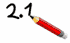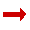# Multiplying Decimals

Multiply without the decimal point, then re-insert it in the correct spot!

## How to Multiply Decimals

• Multiply normally, ignoring the decimal points.
• Then put the decimal point in the answer - it will have as many decimal places as the two original numbers combined.

In other words, just count up how many numbers are after the decimal point in both numbers you are multiplying, then the answer should have that many numbers after its decimal point.

### Example: Multiply 0.03 by 1.1

 start with: 0.03 × 1.1 multiply without decimal points: 3 × 11 = 33 0.03 has 2 decimal places, and 1.1 has 1 decimal place, so the answer has 3 decimal places: 0.033See the Multiplying Decimals Animations

## How Does It Work?

Because when you multiply without the decimal point, you are really shifting the decimal point to the right to get it out of the way:

 Original: 1 Move: 2 Moves: 3 Moves: 0.03 × 1.10.3 × 1.13. × 1.13. × 11.

Then we do the (now easy) multiplication:

3. × 11. = 33.

But remember, we did 3 Moves of the decimal point, so we need to undo that:

 3 Moves: 2 Moves: 1 Move: Correct 33.3.30.330.033

Here are some more examples:

### Example: Multiply 0.25 by 0.2

 start with: 0.25 × 0.2 multiply without decimal points: 25 × 2 = 50 0.25 has 2 decimal places, and 0.2 has 1 decimal place, so the answer has 3 decimal places: 0.050

### Example: Multiply 102 by 0.22

 start with: 102 × 0.22 multiply without decimal points: 102 × 22 = 2244 102 has 0 decimal places, and 0.22 has 2 decimal place, so the answer has 2 decimal places: 22.44

## Common Sense

As a final check you can put your "common sense" hat on and think "is that the right size?", because you don't want to pay ten times too much for anything, nor get only one-tenth of what you need!

And that's all.

Just remember: the answer should have the same number of decimal places as are in both the numbers you are multiplying.

Decimal Worksheets